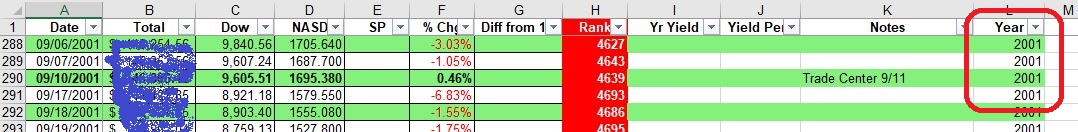# Excel VBA

## Color Rows by Criteria

I have a spreadsheet where the closing value of my portfolio, the NASDAQ, the DJIA, and the S&P close is recorded. See sample below.

Since it is almost 5K rows, I decided I wanted to color the rows with a specific color based on the year.

My list is complete from year 2000 to date (except for a short period where I lost access to some files in a move to new PC).

```Sub ReColorSnapShot()
'ARB 12/02/2020
'+-------------------------Unlock SNAPSHOT,set color scheme by Year, close and report------------------+
'+-----------------------------------------------------------------------------------------------------+
Dim Ranger  As String
Dim LastCol As Long, lastRow As Long
Dim SortRanger As Variant
Dim C   As Long
Dim H   As Long
Dim Quo As String
Dim YearNm As Variant, RGBnm As Variant
Quo = Chr(34)
Application.ScreenUpdating = True
Sheets("SnapShot").Activate
ActiveSheet.Unprotect
lastRow = Range("L99999").End(xlUp).Row
LastCol = 12  'Selection.End(xlToRight).Column
Range(Cells(2, 1), Cells(lastRow, LastCol)).Select
Selection.Interior.ColorIndex = False
Cells(2, 1).Select
YearNm = Array("2000", "2001", "2002", "2003", "2004", "2005", "2006", _
"2010", "2011", "2012", "2013", "2020", "2021")  'omit 1900; make list of years
RGBnm = Array(RGB(153, 204, 255), _
RGB(131, 241, 123), _
RGB(255, 153, 255), _
RGB(255, 255, 0), _
RGB(250, 191, 143), _
RGB(205, 216, 176), _
RGB(153, 204, 0), RGB(204, 255, 204), RGB(102, 204, 255), RGB(255, 204, 153), RGB(204, 192, 218), _
RGB(207, 183, 255), RGB(93, 174, 255))    Each color is full RGB instruction
For H = 0 To UBound(YearNm)	'Read array first to get Year
SortRanger = RGBnm(H)		'Hold RGB current
For C = 2 To lastRow		'Range

If Cells(C, LastCol).Text = YearNm(H) Then	Does year match?
If C Mod 2 = 0 Then		Check whether even numbered row
Sheets("SnapShot").Range(Cells(C, 1), Cells(C, LastCol)).Interior.Color = SortRanger

Else
Sheets("SnapShot").Range(Cells(C, 1), Cells(C, LastCol)).Interior.ColorIndex = False
'No color if odd numbered row
End If
End If
Next C
Next H
Cells(C - 20, 1).Select
ActiveSheet.Protect
MsgBox "Exhausted Year array", vbInformation, "H"
YearNm = 0
RGBnm = 0
C = 0

End Sub
```

In this example some years do not yet have an assigned color. Some of the programming got a little messy. All in all, the initial coding took about an hour and debugging another hour or so.